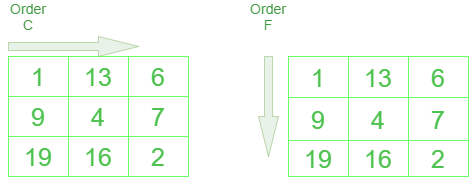Related Articles

# Change the dimension of a NumPy array

• Last Updated : 29 Aug, 2020

Let’s discuss how to change the dimensions of an array. In NumPy, this can be achieved many ways. Let’s discuss each of them.

Method #1: Using Shape()

Syntax :

```array_name.shape()
```

## Python3

 `# importing numpy``import` `numpy as np`` ` ` ` `def` `main():`` ` `    ``# initialising array``    ``print``(``'Initialised array'``)``    ``gfg ``=` `np.array([``1``, ``2``, ``3``, ``4``])``    ``print``(gfg)`` ` `    ``# checking current shape``    ``print``(``'current shape of the array'``)``    ``print``(gfg.shape)``     ` `    ``# modifying array according to new dimensions``    ``print``(``'changing shape to 2,3'``)``    ``gfg.shape ``=` `(``2``, ``2``)``    ``print``(gfg)`` ` `if` `__name__ ``=``=` `"__main__"``:``    ``main()`

Output:

```Initialised array
[1 2 3 4]
current shape of the array
(4,)
changing shape to 2,3
[[1 2]
[3 4]]
```

Method #2: Using reshape()

The order parameter of reshape() function is advanced and optional. The output differs when we use C and F because of the difference in the way in which NumPy changes the index of the resulting array. Order A makes NumPy choose the best possible order from C or F according to available size in a memory block.Difference between Order C and F

Syntax :

```numpy.reshape(array_name, newshape, order= 'C' or 'F' or 'A')
```

## Python3

 `# importing numpy``import` `numpy as np`` ` ` ` `def` `main():`` ` `    ``# initialising array``    ``gfg ``=` `np.arange(``1``, ``10``)``    ``print``(``'initialised array'``)``    ``print``(gfg)``     ` `    ``# reshaping array into a 3x3 with order C``    ``print``(``'3x3 order C array'``)``    ``print``(np.reshape(gfg, (``3``, ``3``), order``=``'C'``))`` ` `    ``# reshaping array into a 3x3 with order F``    ``print``(``'3x3 order F array'``)``    ``print``(np.reshape(gfg, (``3``, ``3``), order``=``'F'``))`` ` `    ``# reshaping array into a 3x3 with order A``    ``print``(``'3x3 order A array'``)``    ``print``(np.reshape(gfg, (``3``, ``3``), order``=``'A'``))`` ` ` ` `if` `__name__ ``=``=` `"__main__"``:``    ``main()`

Output :

```initialised array
[1 2 3 4 5 6 7 8 9]
3x3 order C array
[[1 2 3]
[4 5 6]
[7 8 9]]
3x3 order F array
[[1 4 7]
[2 5 8]
[3 6 9]]
3x3 order A array
[[1 2 3]
[4 5 6]
[7 8 9]]
```

Method #3 : Using resize()

The shape of the array can also be changed using the resize() method. If the specified dimension is larger than the actual array, The extra spaces in the new array will be filled with repeated copies of the original array.

Syntax :

```numpy.resize(a, new_shape)
```

## Python3

 `# importing numpy``import` `numpy as np`` ` ` ` `def` `main():`` ` `    ``# initialise array``    ``gfg ``=` `np.arange(``1``, ``10``)``    ``print``(``'initialised array'``)``    ``print``(gfg)``     ` `    ``# resezed array with dimensions in``    ``# range of original array``    ``np.resize(gfg, (``3``, ``3``))``    ``print``(``'3x3 array'``)``    ``print``(gfg)``     ` `    ``# re array with dimensions larger than``    ``# original array``    ``np.resize(gfg, (``4``, ``4``))``     ` `    ``# extra spaces will be filled with repeated``    ``# copies of original array``    ``print``(``'4x4 array'``)``    ``print``(gfg)``     ` `    ``# resize array with dimensions larger than ``    ``# original array``    ``gfg.resize(``5``, ``5``)``     ` `    ``# extra spaces will be filled with zeros``    ``print``(``'5x5 array'``)``    ``print``(gfg)`` ` ` ` `if` `__name__ ``=``=` `"__main__"``:``    ``main()`

Output :

```initialised array
[1 2 3 4 5 6 7 8 9]
3x3 array
[1 2 3 4 5 6 7 8 9]
4x4 array
[1 2 3 4 5 6 7 8 9]
5x5 array
[[1 2 3 4 5]
[6 7 8 9 0]
[0 0 0 0 0]
[0 0 0 0 0]
[0 0 0 0 0]]
```

Attention geek! Strengthen your foundations with the Python Programming Foundation Course and learn the basics.

To begin with, your interview preparations Enhance your Data Structures concepts with the Python DS Course. And to begin with your Machine Learning Journey, join the Machine Learning – Basic Level Course

My Personal Notes arrow_drop_up